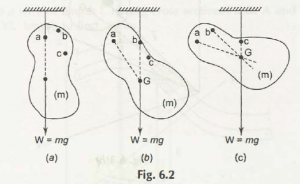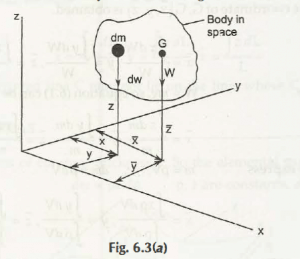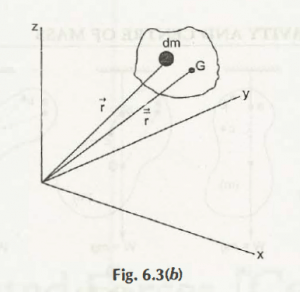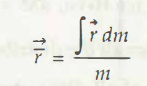With reference to figure 6.2 the body of mass is stopped by the cord which are not extended from point a. The W is denoted by W = mg which will act on vertical basis moving downward. A dotted line is drawn by us from point a moving downwards, and then the body is separated from the point c respectively. All the lines which are drawn vertically meet at one fixed point in above figure b and c at point G which is called Center of Gravity.

In order to calculate center of gravity in mathematical term we should move for fig 6.3 a and bBy moving downward side, in order to find dW in y axis we will see

dM = x dW

Or it can be taken as  M = ʃ x dW for all other distributed forces

In order to get the result about W which lies in y axis we will come across with

M1  =xW

According toVarignon’s theorem (Principle of moments) we can write

M1 =M

x W = ʃ x dW= x dW / W

Same as we can also write the expression for y and z as follows

Y = ʃ y dW /W , z =  ʃ z dW /W

Thus, the coordinate of G, G (x, y,z) is obtained.

x = ʃ x dW /W , y = ʃ y dW /W ,z = ʃ z dW/W

We have

W = mg, so equation (6.1) can be rewritten as

x = ʃ x dm /m , y = ʃ y dm /m ,z = ʃ z dm/m

Now we express

m= Pv ,           dm = pdV

x = ʃ xpdV / ʃ p dV,  y = ʃ y dV / ʃ p dV , z = ʃ z dV / ʃ p dV

Equation (6.2) may be described in vector form (Refer Fig. 6.3(b))Equations (6.2), (6.3) and (6.4) have no reference about ‘g’ (gravitational effect). Therefore, we can come across with unique point (X, y, Z) which is called as the centre of mass. The centre of mass interlinked with the centre of gravity as long as g remain constant.

Links of Next Mechanical Engineering Topics:-### Customer Reviews

My Homework Help
Rated 5.0 out of 5 based on 510 customer reviews at